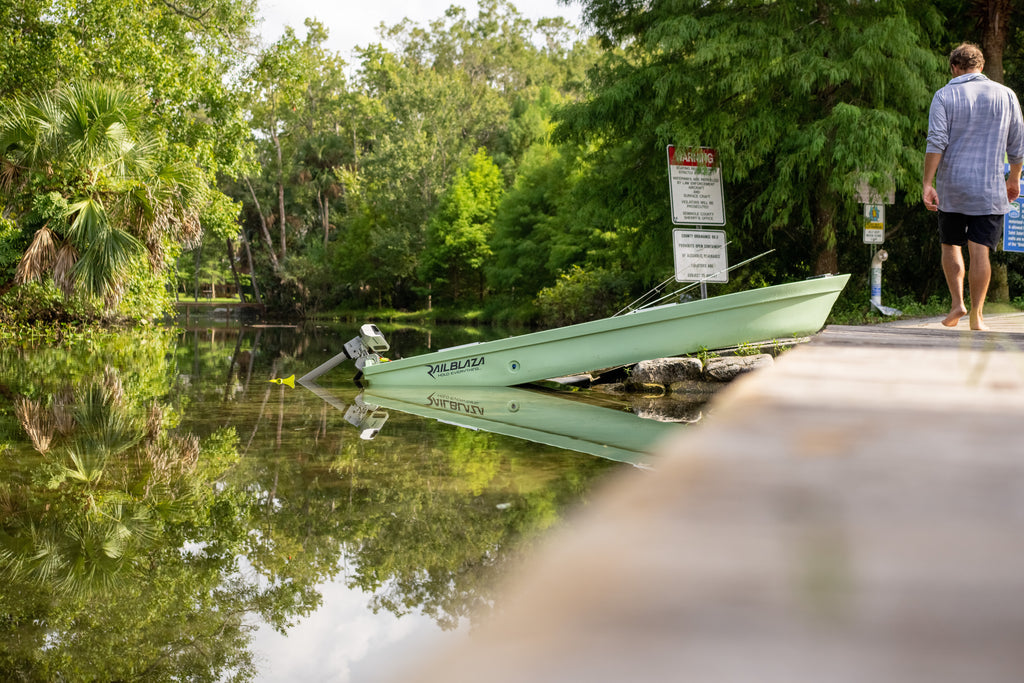# NT300How to Calculate Run Time and Range

The easiest way to understand your electric outboard range and run time is by breaking down and understanding the number of watts your battery capacity is. The 36V 30AH battery we offer is a 30AH which is equivalent to 1200 watt-hours. When the NT300 is in use, the controller displays the watts you are drawing in that specific speed setting. If you are at 50% throttle, the reading should be roughly 600W, and then you can determine your speed by looking at your GPS. At 50% throttle, the GPS reads 5 mph. Now that we have all of this information, we can calculate and estimate our run time and range.

EXAMPLE CALCULATION

1200W - Battery Capacity

600 W - motor running at 50%
5 mph - reading from GPS

To Calculate Range:
Range = Run time x MPH

Run time = 1200Watt hours / 600Watts
Run time = 2 hours

Range = 5 Miles X 2 Hours

Hours

Range = 10 Miles

This calculation shows that with a 1200W